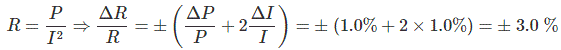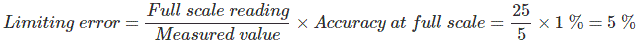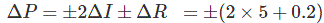# MCQs on Electrical And Electronics Measurements

##### Page 16 of 19. Go to page 1 2 3 4 5 6 7 8 9 10 11 12 13 14 15 16 17 18 19
01․ A 150 V moving iron voltmeter of accuracy class 1-0 reads 75 V when used in a circuit under standard condition. The maximum possible percentage error in the reading is
1%
1.5%
2%
2.5%

Class 1-0 means guaranteed accuracy of 1% of full scale. ± 1 % of 150 volt is ± 1.5v Now, 75 × (L.E./100) = ±1.5 (Limiting Error) L.E. = 2 %

02․ The maximum percentage error in the sum of two voltage measurements when V1 = 100V ± 1% and V2 = 80V ± 5% is
± 4%
± 2.8%
± 6%
± 3%

Given, V1 = 100V ± 1%............. (i) 1% of 100 V is 1 V So, we can rewrite (i) as V1 = 100 V ± 1 V…………(iii) Given, V2 = 80 V ± 5 %............(ii) 5% of 80 V is 4 V So we can rewrite (ii) as V2 = 80 V ± 4 V………….(iv) Now adding (iii) and (iv) V1 + V2 = 180 V ± 5 V……….(v) As, 5 V is 2.8% of 180 V, (v) can be rewritten as V1 + V2 = 180 V ± 2.8%

03․ A resistance of 105 ohms is specified using significant figures as indicated below : 1. 105 ohms 2. 105.0 ohms 3. 0.000105 mega ohms Among these,
1 represents greater precision than 2 and 3.
1, 2 and 3 represent same precision.
2 and 3 represent greater precision than 1.
2 represents greater precision but 1 and 3 represent same precision.

Precision indicates measure of reproducibility of a machine i.e. how nearer the all outputs are for the same inputs. We know, 0.000105 mega ohms = 105 ohms so we can say 1 and 3 has same precision. In case of 105.0 ohms, it has an extra digit after decimal point which leads it to have greater degree of reproducibility. SO, we can say it has greater precision than 1 and 3.

04․ In flux meter, the controlling torque is produced by
weight attached to the moving coil.
spring.
not provided at all.
crossed coil mechanism.

Flux meter is a special type of ballistic galvanometer provided with a heavy electromagnetic damping and very small controlling torque.

05․ The resistance of a circuit is measured from the relation R= P/I2, where P is the power fed into the circuit and I is the current flowing through it. If the limiting errors in the measurement of power and current are respectively ± 1.0% and ± 1.0%, then what will be the limiting error in measurement of resistance ?
± 3.0%
±0.5%
± 1.5%
±3.5%06․ Assertion (A) : Precision is a necessary prerequisiteof accuracy. Reason (R) : Precision guarantees accuracy.
A is true but R is false
A is false bur R is true
Both A and R are true and R is the correct explanation of A
Both A and R are true but R is NOT the correct explanation of A

Accuracy indicates how close the measured value is, with the actual value and precision indicates how close all outputs of the instrument are, for the same input. On the basis of the significance of these two terms we can conclude that precision has nothing to do with accuracy but to attain a good accuracy an instrument must have greater degree of closeness in all results for the same inputs i.e precision is mandatory to have greater accuracy. So we can say Precision is a necessary prerequisite of accuracy but precision does not guarantee accuracy.

07․ An ammeter of range 0-25 A has a guaranteed accuracy of 1% of full-scale reading. The current measured by the ammeter is 5 A. The limiting error in the reading is
3%
4%
5%
6%08․ In a wheatstone bridge, a change of 6 Ω in the unknown arm of bridge is required to produce a change in deflection of 2.4 mm of the galvanometer. The sensitivity is
2.5 mm/Ω
0.8 mm/Ω
0.4 mm/Ω
5.0 mm/Ω

Sensitivity is nothing but the ratio of change in output to change in input. Hence, Sensitivity =2.4/6 = 0.4 mm/Ω.

09․ For a circuit given that I = 2 ± 5 % A, R = 100 ± 0.2 % Ω the limiting error in the power dissipation I2 R in the resistor R is
25.2%
1.2%
5.2%
10.2%

Power dissipation P=I2R10․ A transducer measures a range of 0 – 200 N force with a resolution of 0.15 percent of full scale. What is the smallest change which can be measured by this transducer ?
0.15 N
3.33 N
0.3 N
6.66 N

Resolution is nothing but smallest change in the input that can be measured by the instrument. It is given that Resolution = 0.15 % of full scale. Hence, Smallest change which can be measured by the transducer is (0.15/100) × 200 = 0.3 N.

<<<1415161718>>>